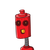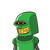# the length of two sides of a triangle are 12 cm and 15 cm. between what two measures should the length of 3rd side?​

the length of two sides of a triangle are 12 cm and 15 cm. between what two measures should the length of 3rd side?​

### 2 thoughts on “the length of two sides of a triangle are 12 cm and 15 cm. between what two measures should the length of 3rd side?​”

1.by Pythagoras we have the min limit of third side as

Let the length of third side be L

15-1215−12 = 3 cm

and we know the sum of any two sides > the third side so 12 + 15 = 27 cm

so the third side ( L) must lie between

3 < L < 27 cm

#shilpi

2.